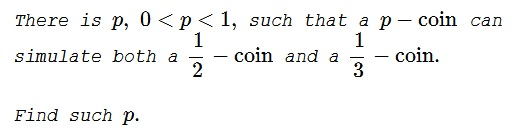# Simulating Probabilities

### Introduction

Call a biased coin a $p-\text{coin}$ $(0\lt p\lt 1)$ if it comes up heads with probability $p$ and tails with probability $1-p.$ We say that $p$ simulates $q$ if by flipping a $p-\text{coin}$ repeatedly (some finite number of times) one can simulate the behavior of a $q-\text{coin}.$ More precisely: there is a positive integer $n$ and a subset of the $2^n$ possible outcomes of flipping the $p-\text{coin}$ $n$ times such that the probability of a sequence of $n$ tosses is in the subset is $q.$ In other words, the experiment is required to terminate in a finite $(n)$ number of cin tosses.

For example, a fair coin can be used to simulate a $\displaystyle \frac{3}{4}-\text{coin}$ by using two flips and defining a pseudo-head to be any two-flip sequence with at least one real head. The chance of a pseudo-head coming up is $\displaystyle \frac{3}{4},$ and so we have simulated a $\displaystyle \frac{3}{4}-\text{coin}.$

### Problem### Solution

It's not hard to see that if $p$ simulates $\displaystyle \frac{1}{2}$ and $\displaystyle \frac{1}{3}$, it must simulate $\displaystyle \frac{1}{6}.$ These are separate problems to show that $\displaystyle p=\frac{1}{6}$ does not work, because it simulates neither $\displaystyle \frac{1}{2}$ nor $\displaystyle \frac{1}{3}.$ This leads to trying to find a $p$ that produces the sequence $HT$ with probability $\displaystyle \frac{1}{6}.$ Such $p$ satisfies $p(1-p)=\displaystyle \frac{1}{6},$ or $6p^2-6p+1=0.$ Let's try $\displaystyle p=\frac{3+\sqrt{3}}{6}.$ This $p$ clearly simulates $\displaystyle \frac{1}{3},$ because the event $\{HT,TH\}$ has the probability $\displaystyle \frac{1}{6}+\frac{1}{6}=\frac{1}{3}.$

Additionally, the event $\{HHH,TTT\}$ has the probability $\displaystyle \frac{1}{2}.$ Indeed,

$\displaystyle p^3+(1-p)^3=\frac{9+5\sqrt{3}}{36}+\frac{9-5\sqrt{3}}{36}=\frac{1}{2}.$

It is obvious that $\displaystyle p=\frac{3-\sqrt{3}}{6}$ also solves the problem.

### $\displaystyle \mathbf{\frac{1}{6}}$ does not simulate $\displaystyle \mathbf{\frac{1}{3}}$

Assume such a simulation possible and that it consists of a subset of $n$ tosses of a $\displaystyle \frac{1}{6}\text{-coin}$, $n\gt 1.$ The probability of an elementary event that consists of $k,$ $0\le k\le n$ heads equals $\displaystyle \frac{5^{n-k}}{6^n}$ so that the numerator of any combination of these probabilities will be either divisible by $5$ or leave the remainder of $1$ when divided by $5.$ In other words, the probability of any event $A$ which is the subset of the sample space of $n$ tosses, $\displaystyle p(A)=\frac{N}{6^n},$ where $N\equiv 0\,(\text{mod}\,5)$ or $N\equiv 1\,(\text{mod}\,5).$

Assuming $N=5k$ and $\displaystyle \frac{N}{6^n}=\frac{1}{3},$ we get $15k=6^{n}$ which is absurd because the left-hand side ends either with $0$ or $5,$ whereas the right-hand side always ends with $6.$ $N=5k+1$ leads to $15k+3=6^{n}$ which is also absurd because the left-hand side ends with either $3$ or $8,$ never with $6.$

### $\displaystyle \mathbf{\frac{1}{6}}$ does not simulate $\displaystyle \mathbf{\frac{1}{2}}$

Similar to the case of $\displaystyle \frac{1}{3},$ we obtain a contradiction from $10k=6^{n}$ or $10k+2=6^{n}.$

### No rational number simulates both $\displaystyle \mathbf{\frac{1}{2}}$ and $\displaystyle \mathbf{\frac{1}{3}}.$

We'll show that no rational number can simulate $\displaystyle \frac{1}{2}.$ (This is also true concerning $\displaystyle \frac{1}{3}$ but is not needed to establish our claim.)

Suppose $\displaystyle p = \frac{j}{k}$ is a rational number, with $j$ and $k$ relatively prime, and $p$ is different from and simulates $\displaystyle \frac{1}{2}.$ This means that there is a positive integer $n$ and integers $\displaystyle a_i,$ $0 \le a_i\le {n\choose i},$ such that

$\displaystyle \sum_{i=0}^na_ip^i(1-p)^{n-i}=\frac{1}{2}.$

Define $\displaystyle b_i={n\choose i}-a_a.$ Observe that, by the binomial theorem,

$\displaystyle \sum_{i=0}^n(a_i+b_i)p^i(1-p)^{n-i}=1.$

In particular, $a_0+b_0=1,$ meaning that either $a_0=0$ or $b_0=0.$ WLOG, assume the former. Then

\displaystyle \begin{align} \frac{1}{2}&=\sum_{i=1}^na_ip^i(1-p)^{n-i}=p\sum_{i=1}^na_{i-1}p^i(1-p)^{n-i}\\ &=\frac{j}{k}\cdot\frac\sum_{i=1}^nj^{i-1}(k-j)^{n-i}}{k^{n-1}}. \end{align

It follows that $\displaystyle k^n=2j\cdot\sum_{i=1}^{n}a_ij^{i-1}(k-j)^{n-i},$ so that $j|k^n.$ Since $j$ and $k$ were assumed to be relatively prime, necessarily $j=1.$ But now note that $\displaystyle 1-p=\frac{k-k}{k}=\frac{k-1}{k}$ also simulates $\displaystyle \frac{1}{2},$ and, by the same reasoning, $k-1=1,$ i.e., $k=2$ and $\displaystyle \frac{1}{2},$ contrary to our assumption.

### Acknowledgment

This is the problem 187 from book Which Way Did The Bicycle Go? by Konhauser, Velleman, Wagon.

The proofs that $\displaystyle \frac{1}{6}$ does not simulate either $\displaystyle \frac{1}{3}$ or $\displaystyle \frac{1}{2}$ follow a suggestion by Sriram Natarajan.

The proof in the last section was adapted from an article Versatile Coins by Istvan Szalkai and Dan Velleman, Am Math Monthly, 100 (1993), 26-33.